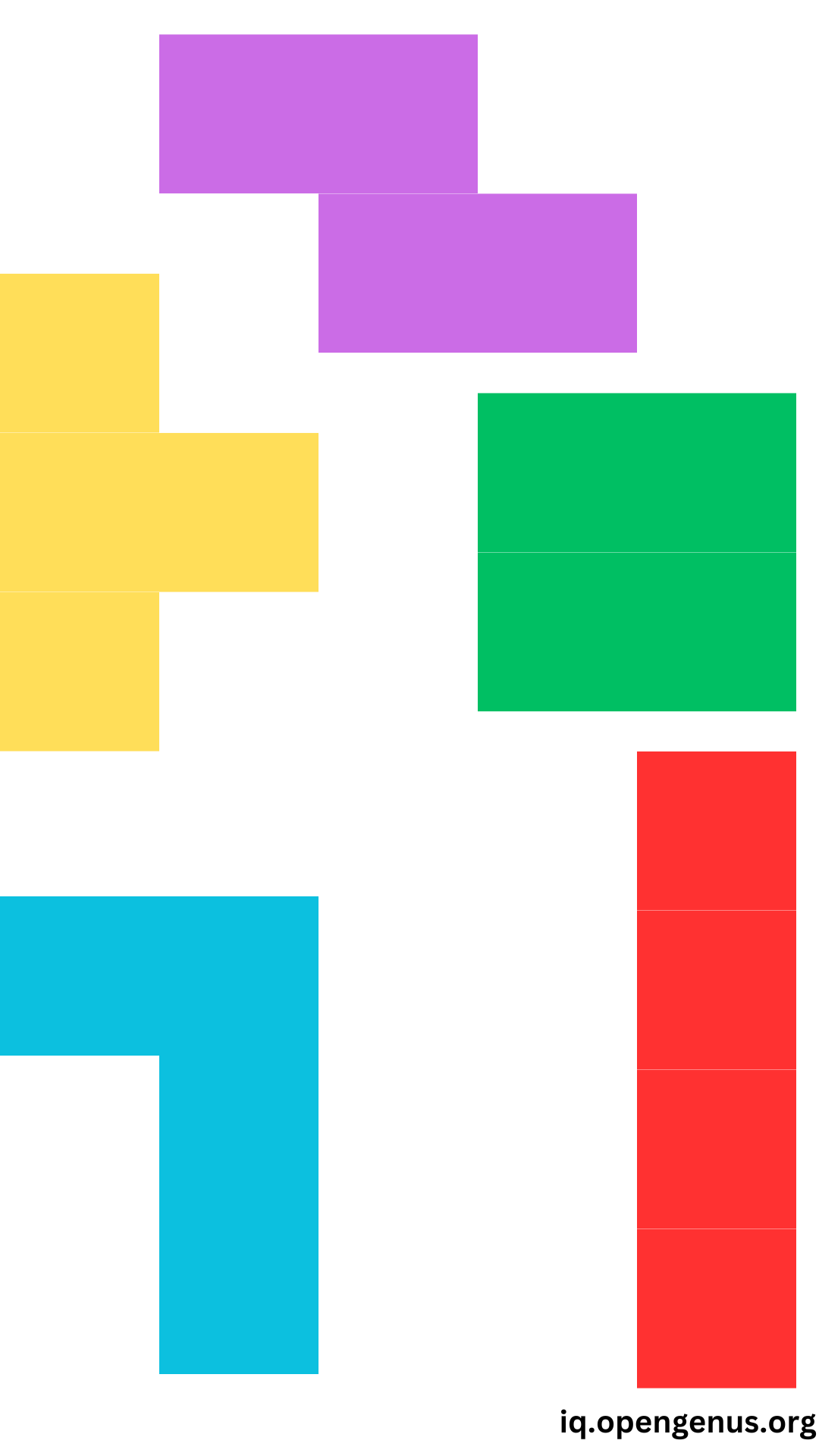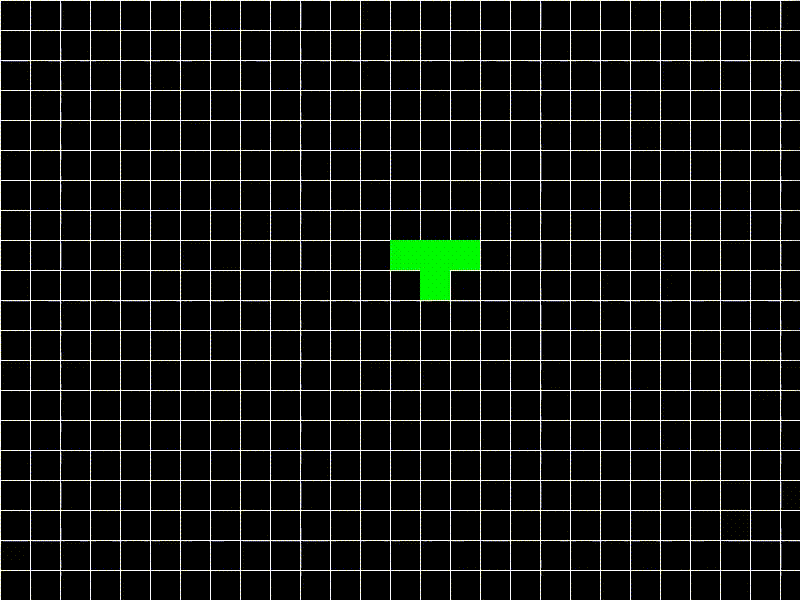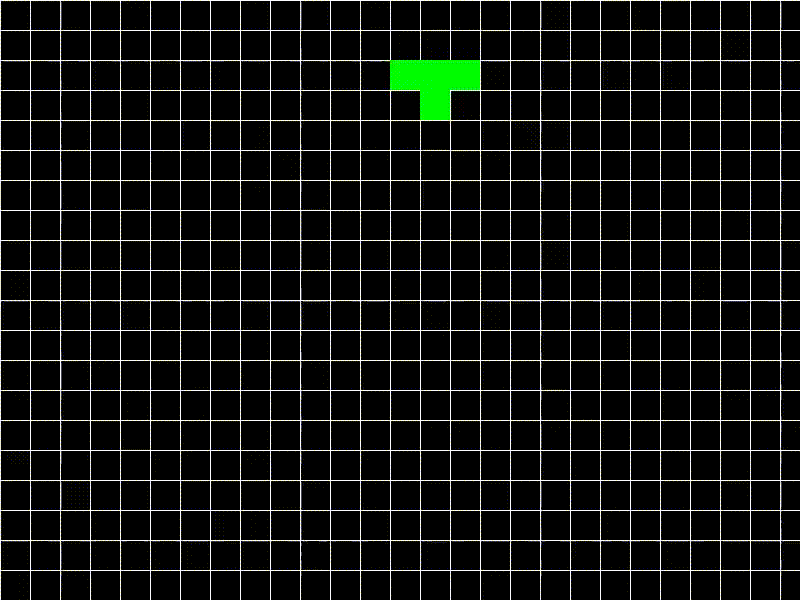×

Search anything:

# Tetris in Python using Pygame [project]

#### PythonGet this book -> Problems on Array: For Interviews and Competitive Programming

In this article at OpenGenus, we will explore the implementation of Tetris game in Python using pygame library. Pygame is a powerful and popular open-source library for developing games using the Python programming language.

1. Introduction
2. Rules
3. Design
4. Implementation
5. Quiz

# Introduction

Tetris is an iconic puzzle game developed by Alexey Pajitnov and released in 1984. The game starts as random coloured letter pieces (geometric shapes) starts falling from the above and can be rotated and aligned before they descend to the bottom and hence the next piece starts falling and goes on.

# Rules

• The game consists of different Tetriminos, which are composed of four square blocks arranged in various shapes. Each Tetrimino falls vertically from the top of the game area.• The Tetriminos can be moved left or right using the arrow keys. They can be rotated using the up arrow key.
• The Tetriminos will fall automatically at a regular interval at the defined speed. The down arrow key can used to make the Tetrimino fall faster.• When a horizontal line is completely filled without any gaps, it disappears, and any blocks above it will fall to fill the gap.
• Points are alotted everytime a line gets cleared.
• The game ends when the Tetriminos stack up to reach the top of the game area.# Design

The Tetris game is designed with a grid-based layout where tetrominoes fall from the top of the screen. The goal is to arrange the tetrominoes in such a way that complete horizontal rows are formed, which will then be cleared from the grid.

• `Grid`: The game is played on a grid, which is represented by a 2D list called grid. Each element of the grid represents a cell and can be empty (0) or occupied by a block (1).

• `Tetromino Shapes`: The tetromino shapes are defined in the PIECES list. Each tetromino shape is represented by a nested list of 1s and 0s, where 1 represents a square of the tetromino and 0 represents an empty space.

• `Movement`: The player can move the currently falling tetromino horizontally using the left and right arrow keys. The tetromino can also be rotated clockwise by pressing the up arrow key. The down arrow key increases the falling speed of the tetromino.

• `Collision Detection`: The check_collision function checks if the current position of the tetromino collides with the boundaries of the grid or with existing blocks in the grid. If a collision is detected, the tetromino's movement in that direction is canceled.

• `Merging Tetrominoes`: When a tetromino reaches its resting position, it merges with the existing blocks in the grid. The merge_tetromino function updates the grid by setting the corresponding cells to 1.

• `Completing Rows`: After a tetromino merges with the grid, the remove_completed_rows function checks if any rows are completely filled with blocks. If so, those rows are removed from the grid, and the above rows are shifted down to fill the empty space.

• `Randomization`: The code uses the random module to select a random tetromino shape and color for the next falling tetromino.

• `Game Over`: If a new tetromino collides with the initial position (which means the game grid is already occupied), the game ends, and the game over screen is displayed.

• `Display and Timing`: The game window is updated using the pygame.display.flip() function. The clock controls the frame rate of the game, and the tick rate can be adjusted to control the speed of the falling tetromino.

• `Score and Time`: The score variable keeps track of the player's score, which increases when rows are cleared. The elapsed time is calculated using pygame.time.get_ticks() and displayed on the game over screen.

Overall, the design of the game focuses on the core mechanics of Tetris, including tetromino movement, collision detection, merging, row clearing, randomization, and score tracking.

# Implementation

## Initial Configuration

``````import pygame
import random
pygame.init()

BLACK = (0, 0, 0)
WHITE = (255, 255, 255)
RED = (255, 0, 0)
GREEN = (0, 255, 0)
BLUE = (0, 0, 255)
CYAN = (0, 255, 255)
MAGENTA = (255, 0, 255)
YELLOW = (255, 255, 0)
ORANGE = (255, 165, 0)
SCREEN_WIDTH = 800
SCREEN_HEIGHT = 600
GRID_SIZE = 30
GRID_WIDTH = SCREEN_WIDTH // GRID_SIZE
GRID_HEIGHT = SCREEN_HEIGHT // GRID_SIZE
INITIAL_POSITION = (GRID_WIDTH // 2, 0)
PIECES = [
[[1, 1, 1, 1]],
[[1, 1], [1, 1]],
[[1, 1, 0], [0, 1, 1]],
[[0, 1, 1], [1, 1, 0]],
[[1, 1, 1], [0, 1, 0]],
[[1, 1, 1], [0, 0, 1]],
[[1, 1, 1], [1, 0, 0]]
]

screen = pygame.display.set_mode((SCREEN_WIDTH, SCREEN_HEIGHT))
pygame.display.set_caption("Tetris")
clock = pygame.time.Clock()
``````
• The code imports the Pygame library and the random module, and then initializes the Pygame modules.
• The code sets various color constants using RGB values.
• Then it sets up constants for screen size, grid size etc.,
• This PIECES list contains the different shapes of Tetriminos represented as nested lists of 1s and 0s. Each inner list represents a row of the shape, and the 1s indicate filled blocks.
• Finally, it sets pygame window with title and creates a clock object.

## Functions

``````def draw_grid():
for x in range(0, SCREEN_WIDTH, GRID_SIZE):
pygame.draw.line(screen, WHITE, (x, 0), (x, SCREEN_HEIGHT))
for y in range(0, SCREEN_HEIGHT, GRID_SIZE):
pygame.draw.line(screen, WHITE, (0, y), (SCREEN_WIDTH, y))

def draw_tetromino(tetromino, position):
for y in range(len(tetromino)):
for x in range(len(tetromino[y])):
if tetromino[y][x] == 1:
pygame.draw.rect(screen, tetromino_color, (position * GRID_SIZE + x * GRID_SIZE,  position * GRID_SIZE + y * GRID_SIZE, GRID_SIZE, GRID_SIZE))

def check_collision(tetromino, position, grid):
for y in range(len(tetromino)):
for x in range(len(tetromino[y])):
if tetromino[y][x] == 1:
if position + x < 0 or position + x >= GRID_WIDTH or \
position + y >= GRID_HEIGHT or grid[position + y][position + x]:
return True
return False

def merge_tetromino(tetromino, position, grid):
for y in range(len(tetromino)):
for x in range(len(tetromino[y])):
if tetromino[y][x] == 1:
grid[position + y][position + x] = 1

def remove_completed_rows(grid):
completed_rows = []
for y in range(GRID_HEIGHT):
if all(grid[y]):
completed_rows.append(y)
for row in completed_rows:
del grid[row]
grid.insert(0,  * GRID_WIDTH)
return len(completed_rows)
``````
• `draw_grid()`: This function is responsible for drawing the grid lines on the game screen. It uses two for loops to draw horizontal and vertical lines, creating a grid-like appearance. The lines are drawn using the pygame.draw.line() function.
• `draw_tetromino(tetromino, position)`: This function is used to draw a Tetromino on the game screen. It takes the tetromino (2D list) and position (current position) as parameters. The function iterates over each element of the Tetromino shape and, if the element is 1, draws a rectangle on the screen using pygame.draw.rect().
• `check_collision(tetromino, position, grid)`: This function checks for collisions between a Tetromino and the grid or other placed Tetrominos. It takes the tetromino, position, and grid as parameters. The function iterates over each element of the Tetromino shape and checks if it intersects with any filled grid cells or extends beyond the grid boundaries.
• `merge_tetromino(tetromino, position, grid)`: This function merges a Tetromino with the existing grid by updating the grid cells where the Tetromino is placed. It takes the tetromino, position, and grid as parameters. The function iterates over each element of the Tetromino shape and updates the corresponding grid cells to 1, indicating that they are filled.
• `remove_completed_rows(grid)`: This function checks for completed rows in the grid (rows that are fully filled) and removes them. It takes the grid as a parameter. The function iterates over each row of the grid and checks if all cells are filled.

## Initialization

``````grid = [ * GRID_WIDTH for _ in range(GRID_HEIGHT)]
tetromino = random.choice(PIECES)
tetromino_color = random.choice([CYAN, YELLOW, MAGENTA, GREEN, RED, BLUE, ORANGE])
position = list(INITIAL_POSITION)
score = 0
start_time = pygame.time.get_ticks()

game_over = False
``````
• `grid`: This variable represents the game grid, which is a 2D list of 0s initially.

• `tetromino`: This variable stores the currently active Tetromino shape chosen randomly from the PIECES list.

• `tetromino_color`: This variable holds the color of the current Tetromino.

• `position`: This variable represents the current position of the Tetromino on the grid.

• `score`: This variable keeps track of the player's score.

• `start_time`: This variable stores the starting time of the game using pygame.time.get_ticks().

• `game_over`: This variable indicates the game state.

## Game Loop

``````while not game_over:
for event in pygame.event.get():
if event.type == pygame.QUIT:
game_over = True

if event.type == pygame.KEYDOWN:
if event.key == pygame.K_LEFT:
position -= 1
if check_collision(tetromino, position, grid):
position += 1
elif event.key == pygame.K_RIGHT:
position += 1
if check_collision(tetromino, position, grid):
position -= 1
elif event.key == pygame.K_DOWN:
position += 1
if check_collision(tetromino, position, grid):
position -= 1
elif event.key == pygame.K_UP:
rotated_tetromino = list(zip(*reversed(tetromino)))
if not check_collision(rotated_tetromino, position, grid):
tetromino = rotated_tetromino

position += 1
if check_collision(tetromino, position, grid):
position -= 1
merge_tetromino(tetromino, position, grid)
completed_rows = remove_completed_rows(grid)
score += completed_rows
tetromino = random.choice(PIECES)
tetromino_color = random.choice([CYAN, YELLOW, MAGENTA, GREEN, RED, BLUE, ORANGE])
position = list(INITIAL_POSITION)
if check_collision(tetromino, position, grid):
game_over = True

screen.fill(BLACK)
draw_grid()
draw_tetromino(tetromino, position)
for y in range(GRID_HEIGHT):
for x in range(GRID_WIDTH):
if grid[y][x] == 1:
pygame.draw.rect(screen, WHITE, (x * GRID_SIZE, y * GRID_SIZE, GRID_SIZE, GRID_SIZE))

pygame.display.flip()
clock.tick(2)
``````
• `Event Handling`: The code begins by iterating through the events in the event queue using pygame.event.get(). It checks for two types of events: pygame.QUIT event(close the game window), and pygame.KEYDOWN event(key press).

• `Key Handling`: Inside the pygame.KEYDOWN event check, the code checks which key was pressed. If the left arrow key is pressed, the position of the Tetromino is updated to move it one unit to the left.

• `Gravity and Collision Handling`: After the key handling, the code moves the Tetromino down by incrementing the y-coordinate of position. If a collision is detected, the Tetromino is moved back up one unit and merged with the grid using merge_tetromino().

• `Game Over Handling`: If there is a collision when the Tetromino is at the initial position, indicating that the grid is already occupied, the game_over flag is set to True, ending the game loop.

• `Drawing on the Screen`: The code then clears the screen by filling it with the BLACK color. It draws the grid using draw_grid() and the current Tetromino using draw_tetromino().

• `Updating the Display`: Finally, the display is updated using pygame.display.flip(), and the clock is ticked to control the frame rate of the game using clock.tick(2). The value 2 represents the frames per second.

## Display Score

``````elapsed_time = (pygame.time.get_ticks() - start_time) / 1000
screen.fill(BLACK)
font = pygame.font.Font(None, 36)
score_text = font.render(f"Score: {score}", True, WHITE)
time_text = font.render(f"Time: {elapsed_time} seconds", True, WHITE)
screen.blit(score_text, (SCREEN_WIDTH // 2 - score_text.get_width() // 2, SCREEN_HEIGHT // 2 - 50))
screen.blit(time_text, (SCREEN_WIDTH // 2 - time_text.get_width() // 2, SCREEN_HEIGHT // 2))
pygame.display.flip()
pygame.time.wait(5000)
pygame.quit()
``````
• `Elapsed Time`: The variable elapsed_time is calculated by subtracting the start_time (the starting time of the game) from the current time using pygame.time.get_ticks(). It is divided by 1000 to convert the time to seconds.
• `Clear Screen`: The screen is cleared by filling it with the BLACK color using screen.fill(BLACK). A font object is created using pygame.font.Font() to define the font style and size for the text that will be displayed.
• `Render Text`: The score and elapsed time are rendered as text using the font object. The render() function is called on the font object, passing the text, a boolean value indicating antialiasing, and the color of the text.
• `Update Display`: The display is updated using pygame.display.flip() to show the rendered text on the screen. The program then waits for 5 seconds using pygame.time.wait(5000), pausing the execution for the specified time.
• `Quit Game`: Finally, the Pygame module is quit using pygame.quit() to properly exit the game.

## Solution

``````import pygame
import random
pygame.init()

BLACK = (0, 0, 0)
WHITE = (255, 255, 255)
RED = (255, 0, 0)
GREEN = (0, 255, 0)
BLUE = (0, 0, 255)
CYAN = (0, 255, 255)
MAGENTA = (255, 0, 255)
YELLOW = (255, 255, 0)
ORANGE = (255, 165, 0)
SCREEN_WIDTH = 800
SCREEN_HEIGHT = 600
GRID_SIZE = 30
GRID_WIDTH = SCREEN_WIDTH // GRID_SIZE
GRID_HEIGHT = SCREEN_HEIGHT // GRID_SIZE
INITIAL_POSITION = (GRID_WIDTH // 2, 0)
PIECES = [
[[1, 1, 1, 1]],
[[1, 1], [1, 1]],
[[1, 1, 0], [0, 1, 1]],
[[0, 1, 1], [1, 1, 0]],
[[1, 1, 1], [0, 1, 0]],
[[1, 1, 1], [0, 0, 1]],
[[1, 1, 1], [1, 0, 0]]
]

screen = pygame.display.set_mode((SCREEN_WIDTH, SCREEN_HEIGHT))
pygame.display.set_caption("Tetris")
clock = pygame.time.Clock()

def draw_grid():
for x in range(0, SCREEN_WIDTH, GRID_SIZE):
pygame.draw.line(screen, WHITE, (x, 0), (x, SCREEN_HEIGHT))
for y in range(0, SCREEN_HEIGHT, GRID_SIZE):
pygame.draw.line(screen, WHITE, (0, y), (SCREEN_WIDTH, y))

def draw_tetromino(tetromino, position):
for y in range(len(tetromino)):
for x in range(len(tetromino[y])):
if tetromino[y][x] == 1:
pygame.draw.rect(screen, tetromino_color, (position * GRID_SIZE + x * GRID_SIZE,  position * GRID_SIZE + y * GRID_SIZE, GRID_SIZE, GRID_SIZE))

def check_collision(tetromino, position, grid):
for y in range(len(tetromino)):
for x in range(len(tetromino[y])):
if tetromino[y][x] == 1:
if position + x < 0 or position + x >= GRID_WIDTH or \
position + y >= GRID_HEIGHT or grid[position + y][position + x]:
return True
return False

def merge_tetromino(tetromino, position, grid):
for y in range(len(tetromino)):
for x in range(len(tetromino[y])):
if tetromino[y][x] == 1:
grid[position + y][position + x] = 1

def remove_completed_rows(grid):
completed_rows = []
for y in range(GRID_HEIGHT):
if all(grid[y]):
completed_rows.append(y)
for row in completed_rows:
del grid[row]
grid.insert(0,  * GRID_WIDTH)
return len(completed_rows)

grid = [ * GRID_WIDTH for _ in range(GRID_HEIGHT)]
tetromino = random.choice(PIECES)
tetromino_color = random.choice([CYAN, YELLOW, MAGENTA, GREEN, RED, BLUE, ORANGE])
position = list(INITIAL_POSITION)
score = 0
start_time = pygame.time.get_ticks()

game_over = False
while not game_over:
for event in pygame.event.get():
if event.type == pygame.QUIT:
game_over = True

if event.type == pygame.KEYDOWN:
if event.key == pygame.K_LEFT:
position -= 1
if check_collision(tetromino, position, grid):
position += 1
elif event.key == pygame.K_RIGHT:
position += 1
if check_collision(tetromino, position, grid):
position -= 1
elif event.key == pygame.K_DOWN:
position += 1
if check_collision(tetromino, position, grid):
position -= 1
elif event.key == pygame.K_UP:
rotated_tetromino = list(zip(*reversed(tetromino)))
if not check_collision(rotated_tetromino, position, grid):
tetromino = rotated_tetromino

position += 1
if check_collision(tetromino, position, grid):
position -= 1
merge_tetromino(tetromino, position, grid)
completed_rows = remove_completed_rows(grid)
score += completed_rows
tetromino = random.choice(PIECES)
tetromino_color = random.choice([CYAN, YELLOW, MAGENTA, GREEN, RED, BLUE, ORANGE])
position = list(INITIAL_POSITION)
if check_collision(tetromino, position, grid):
game_over = True

screen.fill(BLACK)
draw_grid()
draw_tetromino(tetromino, position)
for y in range(GRID_HEIGHT):
for x in range(GRID_WIDTH):
if grid[y][x] == 1:
pygame.draw.rect(screen, WHITE, (x * GRID_SIZE, y * GRID_SIZE, GRID_SIZE, GRID_SIZE))

pygame.display.flip()
clock.tick(2)

elapsed_time = (pygame.time.get_ticks() - start_time) / 1000
screen.fill(BLACK)
font = pygame.font.Font(None, 36)
score_text = font.render(f"Score: {score}", True, WHITE)
time_text = font.render(f"Time: {elapsed_time} seconds", True, WHITE)
screen.blit(score_text, (SCREEN_WIDTH // 2 - score_text.get_width() // 2, SCREEN_HEIGHT // 2 - 50))
screen.blit(time_text, (SCREEN_WIDTH // 2 - time_text.get_width() // 2, SCREEN_HEIGHT // 2))
pygame.display.flip()
pygame.time.wait(5000)
pygame.quit()
``````

## Output# Quiz

## Question 1

#### Which arrow key is used to make the Tetrimino fall faster?

Down
Up
Right
Left
The Tetriminos will fall automatically at a regular interval at the defined speed. The down arrow key can used to make the Tetrimino fall faster.

## Question 2

#### Pygame library can be used to develop games using python?

True
False
Pygame is a powerful and popular open-source library for developing games using the Python programming language.